# Line segment

The 4 cm long line segment is enlarged in the ratio of 5/2. How many centimeters will measure the new line segment?

x =  10 cm

### Step-by-step explanation: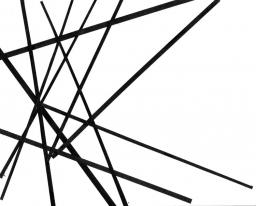Did you find an error or inaccuracy? Feel free to write us. Thank you!Tips to related online calculators
Check out our ratio calculator.

## Related math problems and questions:

• Line segmentCut a line segment of 15 cm into two line segments so that their lengths are in ratio 2:1. What length will each have?
• Line segment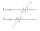Line segment AB is 8 cm long. Divide it in a ratio of 2: 3.
• Divide in ratioLine segment AB 12 cm long divide in a ratio of 5: 3. How long are the individual parts?
• Map 2At what scale is made map if the distance 8.2 km corresponds on the map segment 5 cm long?
• Points on line segmentPoints P & Q belong to segment AB. If AB=a, AP = 2PQ = 2QB, find the distance: between point A and the midpoint of the segment QB.
• Sides od triangleSides of the triangle ABC has length 4 cm, 5 cm and 7 cm. Construct triangle A'B'C' that are similar to triangle ABC which has a circumference of 12 cm.
• Enlarged rectangleThe rectangle with dimensions of 8 cm and 12 cm is enlarged in a ratio of 5:4. What are the circumference and the area of the enlarged rectangle?
• Trapezoid - intersection of diagonalsIn the ABCD trapezoid is AB = 8 cm long, trapezium height 6 cm, and distance of diagonals intersection from AB is 4 cm. Calculate the trapezoid area.
• Dividing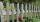Divide the three-line segment 13 cm, 26 cm, and 19.5 cm long for parts so that the individual pieces were equally long and longest. How long will the individual parts, and how many will it?
• 2d shapeCalculate the content of a shape in which an arbitrary point is not more than 3 cm from the segment AB. The length of the segment AB is 5 cm.
• Line segmentFor the line segment whose endpoints are L[-1, 13] and M[18, 2], find the x and y value for the point located 4 over 7 the distance from L to M.
• On the mapA line 1.5 cm long corresponds to a line 3 cm long on the map. What is the scale of the map?
• Slope RR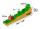A line has a rise of 2 and a run of 11. What is the slope?
• SegmentsLine segments 62 cm and 2.2 dm long we divide into equal parts which lengths in centimeters is expressed integer. How many ways can we divide?
• Photocopier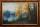A photocopier enlarges a picture in the ratio 7:4. How many times will a picture of size 6cm by 4cm be enlarged to fit on a 30cm by 20 cm page?
• Slope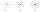Calculate the slope of a line that intersects points (-84,41) and (-76,-32).
• Trapezoid MO-5-Z8ABCD is a trapezoid that lime segment CE is divided into a triangle and parallelogram, as shown. Point F is the midpoint of CE, DF line passes through the center of the segment BE, and the area of the triangle CDE is 3 cm2. Determine the area of the trape